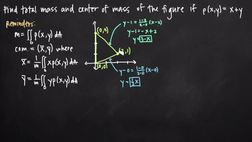PLAY PREVIEW

### Segments in this Video

#### Using Equations to Find Mass and Center of Mass(02:25)

FREE PREVIEW

To find the mass and center of mass of a figure, use the equation for m and the double integral of the equation for rho.

#### The Inner Integral Limits(03:12)

The lower limit of integration with respect to y is going to be defined by the line that defines the lower bound of the region. When the inner integral has limits of integration with respect to y, we must have dy on the inside.

#### Simplifying X and Y(04:16)

We found that the mass of the object is 6 and using that we found the center of mass. We can set up the equations or x bar and y bar. The integrals will have the same limits of integration.

#### Solving for the Center of Mass(01:59)

We will multiply y by the equation for rho of xy and then by dy and dx. This will give us two double integrals. We will use this to solve for the center of mass.

#### Credits(00:00)

Credits

For additional digital leasing and purchase options contact a media consultant at 800-257-5126
(press option 3) or sales@films.com.

# Double integrals to find mass and center of mass

Part of the Series : Integral Calc: Calculus 3
 3-Year Streaming Price: \$49.95

Share

### Description

This video tutorial works through math problems/equations that address topics in Calculus 3, Multiple Integrals. This specific tutorial addresses Double integrals to find mass and center of mass.

Length: 12 minutes

Item#: BVL275689Closed Captioned